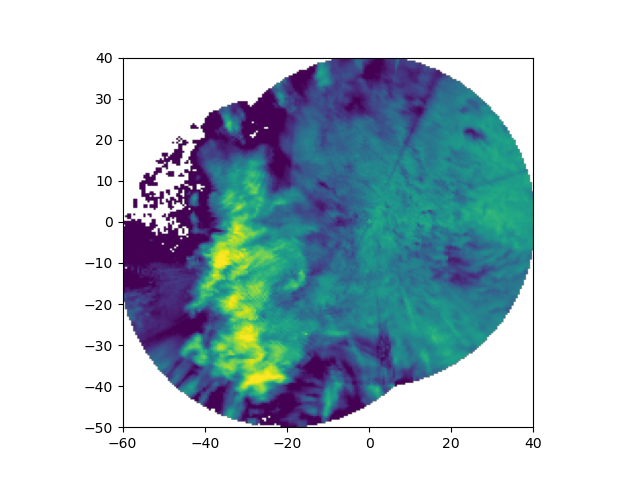# Map two radars to a Cartesian grid#

Map the reflectivity field of two nearby ARM XSARP radars from antenna coordinates to a Cartesian grid.```print(__doc__)

# Author: Jonathan J. Helmus (jhelmus@anl.gov)

import matplotlib.pyplot as plt

import pyart
from pyart.testing import get_test_data

xsapr_sw_file = get_test_data("swx_20120520_0641.nc")
xsapr_se_file = get_test_data("sex_20120520_0641.nc")

# filter out gates with reflectivity > 100 from both radars
gatefilter_se.exclude_transition()
gatefilter_se.exclude_above("corrected_reflectivity_horizontal", 100)
gatefilter_sw.exclude_transition()
gatefilter_sw.exclude_above("corrected_reflectivity_horizontal", 100)

# perform Cartesian mapping, limit to the reflectivity field.
gatefilters=(gatefilter_se, gatefilter_sw),
grid_shape=(1, 201, 201),
grid_limits=((1000, 1000), (-50000, 40000), (-60000, 40000)),
grid_origin=(36.57861, -97.363611),
fields=["corrected_reflectivity_horizontal"],
)

# create the plot
fig = plt.figure()